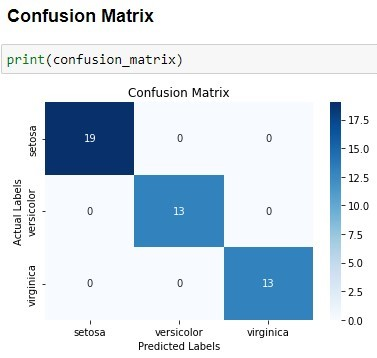# Plotting a Confusion Matrix

A confusion matrix is a useful tool for evaluating the performance of a machine learning algorithm. It is a table that displays the predicted and actual classifications of a set of data, allowing us to determine the accuracy of the model’s predictions. The rows of the confusion matrix represent the actual labels, while the columns represent the predicted labels. The diagonal elements of the confusion matrix represent the number of correct predictions, while the off-diagonal elements represent the number of incorrect predictions.

• Shown below is an example of a confusion matrix using the Iris dataset:#### Importing libraries and creating a pipeline:

For no redundancy in the code snippets below, we’ll first do the necessary steps here. The method is mentioned below:

• Imports necessary libraries such as `pandas`, `NumPy`, `matplotlib`, `seaborn`, `scikit-learn`, `load_iris`, `train_test_split`, `confusion_matrix`, and `DecisionTreeClassifier`.
• The dataset is split into a training set and a testing set using the `train_test_split()` function from `scikit-learn`. The classifier is trained on the training set and used to predict the class labels of the testing set.
• The confusion matrix is calculated using scikit-learn’s `confusion_matrix()` function, which compares the true class labels of the testing set to the predicted class labels.

Now coming onto the different methods that we can use to plot a confusion matrix:

#### 1. Using the "seaborn" library:

• The `seaborn` library is used to visualize the confusion matrix as a heatmap with annotations for each cell. The heatmap provides a visual representation of the confusion matrix, making it easier to identify patterns and trends in the data.
• This solution is by far the simplest methodology to plot a confusion matrix

#### 2. Using the "matplotlib" library:

• The method creates a figure and axis using matplotlib subplots.
• It generates a heatmap of the confusion matrix using the `imshow` function.
• It iterates through the rows and columns of the confusion matrix to add the counts as text to the heatmap.
• It sets the title, x-label, and y-label of the plot.
• It sets the ticks and labels of the x-axis and y-axis to the target names of the iris dataset.
• It adds a color bar to the plot.
• Finally, it displays the plot using the `show` function.
• The solution of this method is comparatively longer than the other two methods.

#### 3. Using the "pandas" library:

• The resulting confusion matrix is converted to a `Pandas DataFrame` and visualized using seaborn’s `heatmap` function. The heatmap displays the true class labels on the y-axis and the predicted class labels on the x-axis.
1 Like